# Chapter 5 Visualizing data in R – An intro to ggplot

These notes accompany portions of Chapter 2 — Displaying Data — of our textbook, which we revisit later. The reading below is required, Whitlock and Schluter (2020) is not.

Motivating scenarios: Motivating scenarios: you have a fresh new data set and want to check it out. How do you go about looking into it?

Learning goals: By the end of this chapter you should be able to:

• Build a simple ggplot.
• Explain the idea of mapping data onto aesthetics, and the use of different geoms.
• Match common plots to common data type.
• Use geoms in ggplot to generate the common plots (above).
There is no external reading for this chapter, but watch the embedded vidoes, and complete all embedded learnR excercises. Then go to canvas to fill out the evaluation. You will need to make three very different types of plots from the mpg data.

## 5.1 A quick intro to data visualization.

Recall that as bio-statisticians, we bring data to bear on critical biological questions, and communicate these results to interested folks. A key component of this process is visualizing our data.

### 5.1.1 Exploratory and explanatory visualizationsWe generally think of two extremes of the goals of data visualization

• In exploratory visualizations we aim to identify any interesting patterns in the data, we also conduct quality control to see if there are patterns indicating mistakes or biases in our data, and to think about appropriate transformations of data. On the whole, our goal in exploratory data analysis is to understand the stories in the data.• In explanatory visualizations we aim to communicate our results to a broader audience. Here our goals are communication and persuasion. When developing explanatory plots we consider our audience (scientists? consumers? experts?) and how we are communicating (talk? website? paper?).

The ggplot2 package in R is well suited for both purposes. Today we focus on exploratory visualization in ggplot2 because

1. They are the starting point of all statistical analyses.
2. You can do them with less ggplot2 knowledge.
3. They take less time to make than explanatory plots.

Later in the term we will show how we can use ggplot2 to make high quality explanatory plots.

### 5.1.2 Centering plots on biology

Whether developing an explanatory or exploratory plot, you should think hard about the biology you hope to convey before jumping into a plot. Ask yourself

• What do you hope to learn from this plot?
• Which is the response variable (we usually place that on the y-axis)?
• Are data numeric or categorical?
• If they are categorical are they ordinal, and if so what order should they be in?

The answers to these questions should guide our data visualization strategy, as this is a key step in our statistical analysis of a dataset. The best plots should evoke an immediate understanding of the (potentially complex) data. Put another way, a plot should highlight both the biological question and its answer.

Before jumping into making a plot in R, it is often useful to take this step back, think about your main biological question, and take a pencil and paper to sketch some ideas and potential outcomes. I do this to prepare my mind to interpret different results, and to ensure that I’m using R to answer my questions, rather than getting sucked in to so much Ring that I forget why I even started. With this in mind, we’re ready to get introduced to ggploting!

## 5.2 An intro to today’s data

So, let’s do this with a data set msleep (click link for more info) built in to tidyverse, which contains interesting information about a bunch of mammals. One question we might have is - how much variability is there in mammal brain size, and what explains this variation? Let’s look to find out.

#### Data prep and transformation

Before making these plots, we’ll use the mutate() function to $$log_{10}$$ transform brainwt and bodywt. For this data set, this makes patterns easier to see. Later we’ll see when this is a good or bad idea and how to do this while we are plotting, but let’s hold off on that for now.

msleep <- msleep %>%
mutate(log10_brainwt = log10(brainwt),
log10_bodywt  = log10(bodywt))

Here are the data to get a sense of it.

## 5.3 The idea of ggplot

Figure 5.1: Watch this video about getting started with ggplot2 (7 min and 17 sec), from STAT 545

ggplot is built on a framework for building plots called the grammar of graphics. A major idea here is that plots are made up of data that we map onto aesthetic attributes.

Lets unpack this sentence, because there’s a lot there. Say we wanted to make a very simple plot e.g. observations for categorical data, or a simple histogram for a single continuous variable. Here we are mapping this variable onto a single aesthetic attribute – the x-axis.

There’s way more nuance / awesomeness / power / ideas behind ggplot2 which we will reveal just in time over the term, but here we get going with the essential concepts. If you want to learn more about ggplot in one place, check out the ggplot2 book and/or the socviz book .

### 5.3.1 Mapping aesthetics to variables

So first we need to think of the variables we hope to map onto an aesthetic. Here, the only variable from the data set we are considering is how many hours an organism is awake per day. This is a continuous variable, which we hope to map on to the x-axis. We do so like this:

ggplot(data = msleep, aes(x = log10_brainwt))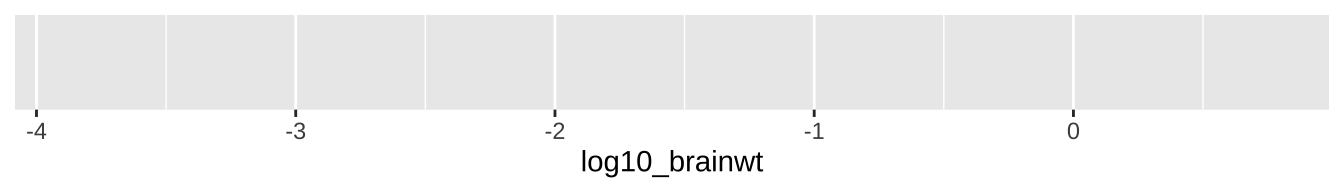Figure 5.2: Uhh ohhh

So, that didn’t go as expected :( — there just a blank grey background. But look more closely, what do you notice?

We make a more useful plot by adding a geom layer.

Adding things to a ggplot. You see that we keep adding to our plots with a + sign. I often accidently use the pipe operator %>% sign used to pipe results from one procedure to the next in dplyr by mistake. R will gently notify you when you make this common error.

geoms explain to R what we want to see: points, lines, histograms etc… In a future chapter, we will see how to add data summaries and trendlines. As we discuss below, a histogram is a great way to visualize variability, so lets add that as a geom.

ggplot(data = msleep, aes(x = log10_brainwt))   +
geom_histogram(bins =10, color = "white")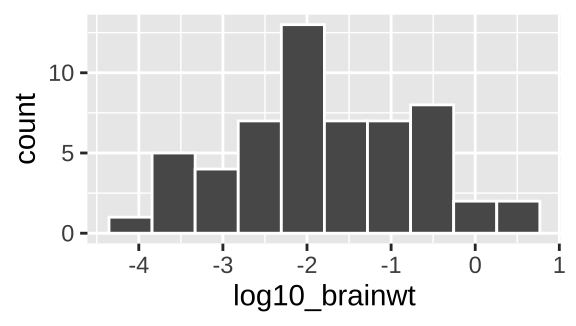Figure 5.3: Our first ggplot!

Yay! OK - we’re off. Below we explore a bunch more geom and how they relate to the type of variables we’re interested in! But first, one more awesome feature of ggplot – faceting.

In the bible of data visualization, the Visual Display of Quantitative Information, Tufte (1983), introduced the idea of “small multiples” as a way to efficiently visualize data in many dimensions, by making many small graphs for different values of categorical variables.

Small multiples are economical: once viewers understand the design of one [chart], they have immediate access to the data in all the other [charts]. […] as the eye moves from one [chart] to the next, the constancy of the design allows the viewer to focus on changes in the data rather than on changes in graphical design.
— Edward Tufte

In ggplot we can build small multiples as facets, with the facet_wrap() and facet_grid() functions.

ggplot(data = msleep, aes(x = log10_brainwt))   +
geom_histogram(bins =10, color = "white") +
facet_wrap(~vore, ncol =3)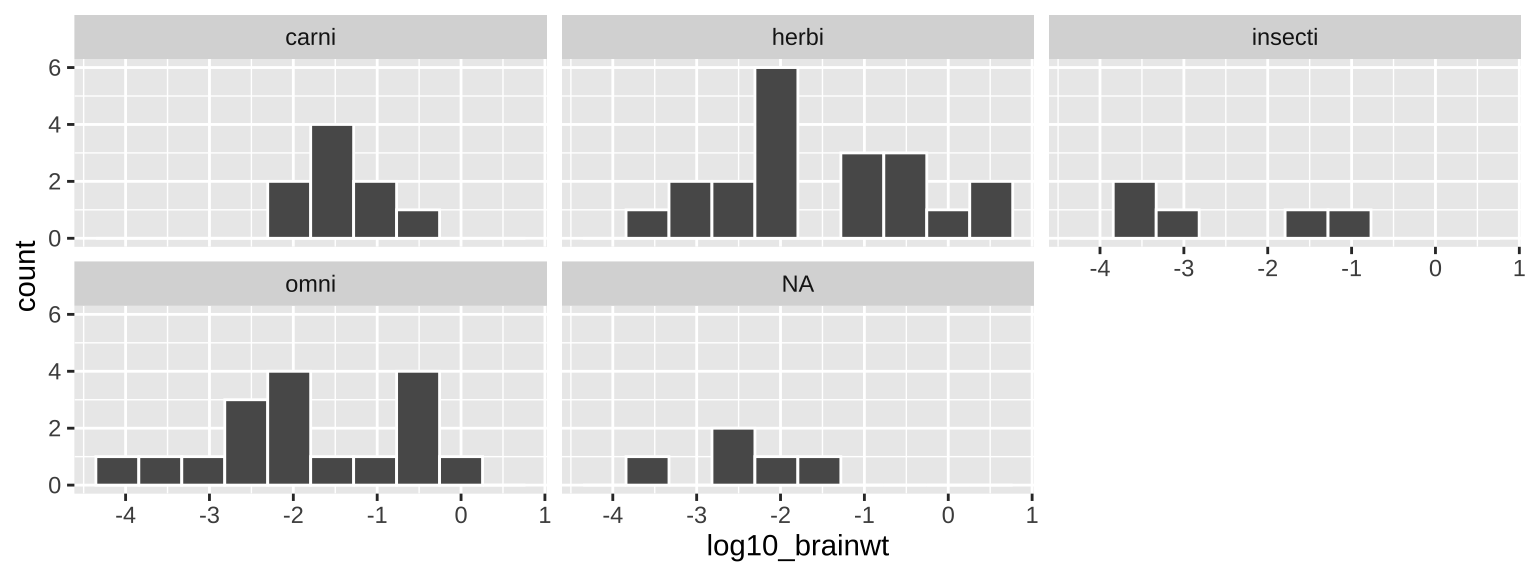Figure 5.4: Our first facetted ggplot!

### 5.3.4 An aside – saving and adding to plots

Above, we made our final faceted figure (5.3) by copying what we coded in our histogram figure (5.3) and adding the facet_wrap() function. Alternatively, we can recreate Fig. 5.4 by saving the plot as we go and adding on as follows:

plot_one <- ggplot(data = msleep, aes(x = log10_brainwt)) +
geom_histogram(bins =10, color = "white")

plot_one +
facet_wrap(~vore, ncol =3) 
## Warning: Removed 27 rows containing non-finite values (stat_bin).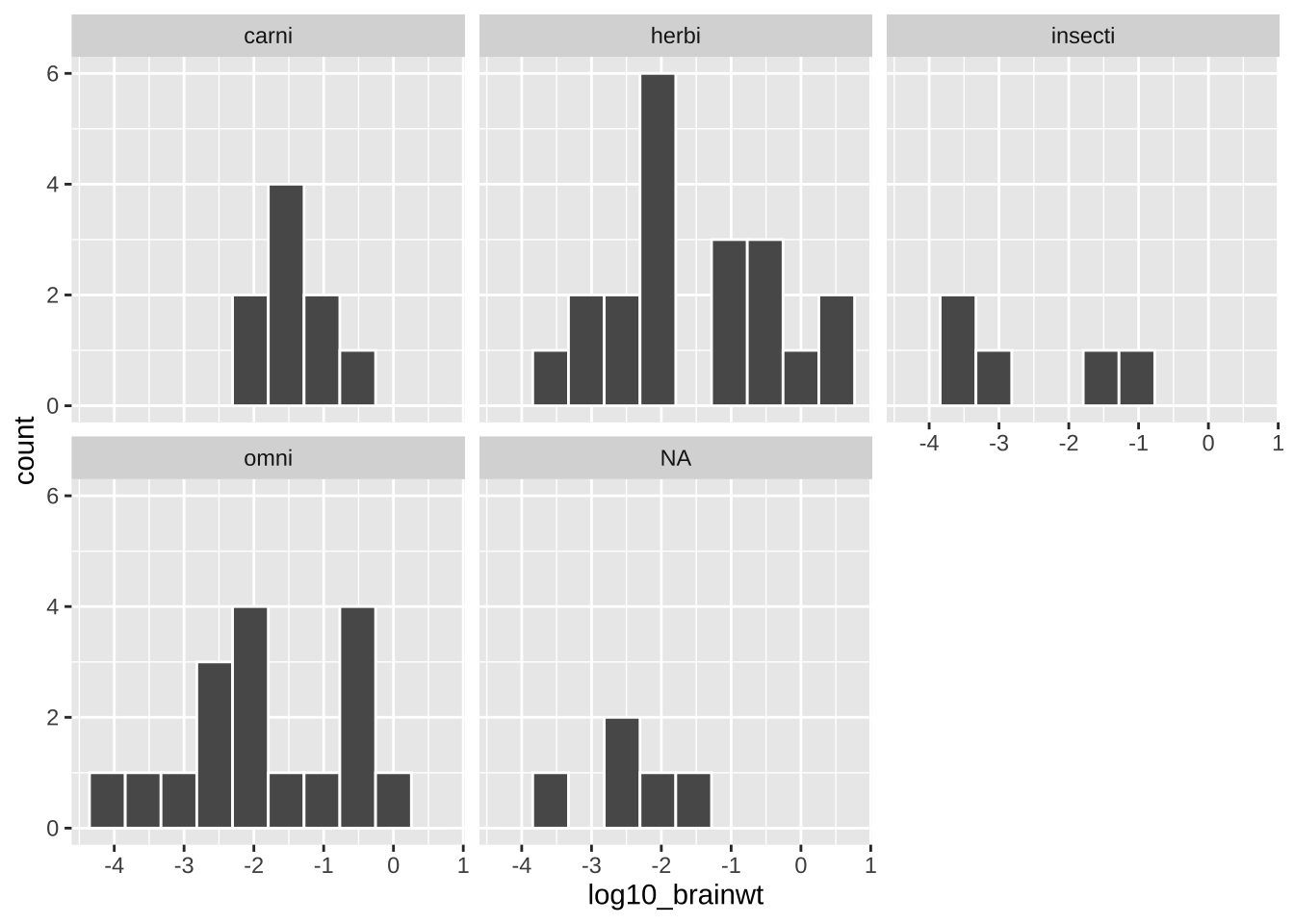It is up to you if you want to save intermediate efforts in a plot.

### 5.3.5ggplot2 primer

To get more of a sense of how to use ggplot, complete the Primer on Data Visualization Basics primer embedded below. This will get you a solid foundation in R which we will build off in this lecture and in the coming weeks.

## 5.4 Common types of plots

As we saw in the section, Centering plots on biology, we want our biological questions and the structure of the data to guide our plotting choices. So, before we get started on making plots, we should think about our data.

• What are the variable names?
• What are the types of variables?
• What are our motivating questions and how do the data map onto these questions?
• Etc…

Using the msleep data set below, we briefly work through a rough guide on how the structure of our data can translate into a plot style, and how we translate that into a geom in ggplot. So the first step you should look at the data – either with the view() function, or a quick glimpse() and reflect on your questions before plotting. This also helps us remember the name and data type of each variable.

glimpse(msleep)

Now we’re nearly ready to get started, but first, some caveats

1. These are vey preliminary exploratory plots – and you may need more advanced plotting R talents to make plots that better help you see patterns. We will cover these in Chapters YB ADD, where we focus on explanatory plots.

2. There are not always cookie cutter solutions, with more complex data you may need more complex visualizations.

That said, the simple visualization and R tricks we learn below are the essential building blocks of most data presentation. So, let’s get started!

There is a lot of stuff below. We will revisit all of it again and again over the term, so you don’t need to master it now – think of this as your first exposure. You’ll get more comfortable and this will become more natural over time.

### 5.4.1 One variable

With one variable, we use plots to visualize the relative frequency (on the y-axis) of the values it takes (on the x-axis).

gg-plotting one variable We map our one variable of interest onto x aes(x = <x_variable>), where we replace <x_variable> with our x-variable. The mapping of frequency onto the y happens automatically.

#### One categorical variable

Say we wanted to know how many carnivores, herbivores, insectivores, and omnivores in the msleep data set. From the output of the glimpse() function above, we know that vore is a categorical variable, so we want a simple bar plot, which we make with geom_bar().

ggplot(data = msleep, aes(x = vore)) +
geom_bar()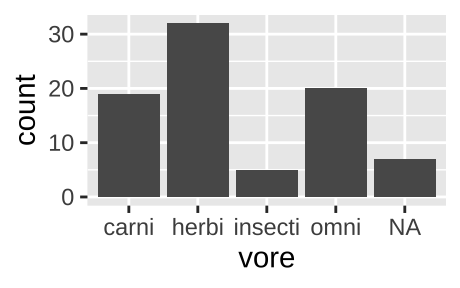Figure 5.6: Classic barplot

We can also pipe data into ggplot argument after doing stuff to the data. For example, the code below remove NA values from our plot.

msleep                 %>%
filter(!is.na(vore)) %>%
ggplot(aes(x = vore)) +
geom_bar()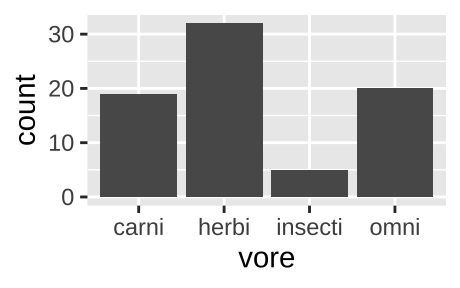Figure 5.7: A barplot, like the one above, but with NA values removed.

If the same data where presented as one categorical variable for vore (with each vore once) and another, n, for counts.

count(msleep, vore)
## # A tibble: 5 × 2
##   vore        n
##   <chr>   <int>
## 1 carni      19
## 2 herbi      32
## 3 insecti     5
## 4 omni       20
## 5 <NA>        7

We could recreate figure 5.6 with geom_col(). again mapping vore to the x-aesthetic, and now mapping count to the y aesthetic, by as follows:

count(msleep, vore) %>%
ggplot(aes(x = vore, y = n))+
geom_col()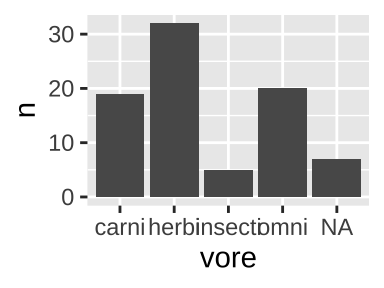#### One continuous variable

We are often interested to know how variable our data is, and to think about the shape of this variability. Revisiting our data on mammal sleep patterns, we might be interested to evaluate the variability in how long mammals sleep.

• Do all species sleep roughly the same amount?
• Is the data bimodal (with two humps)?
• Do some species sleep for an extraordinarily long or short amount of time?

We can look into this with a histogram or a density plot.

##### One continuous variable: A histogram

We use the histogram geom, geom_histogram(), to make a histogram in R.

ggplot(msleep, aes(x = log10_brainwt))+
geom_histogram(bins = 10, color  = "white") # Bins tells R we want 10 bins, and color = white tells R we want white lines between our bins
## Warning: Removed 27 rows containing non-finite values (stat_bin).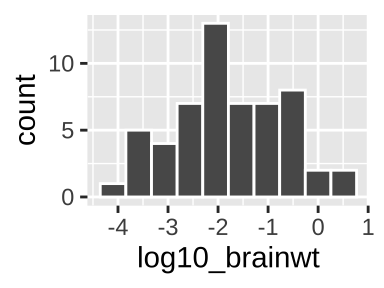In a histogram, each value on the x represents some interval of values of our categorical variable (in this case, we had 10 bins, but we could have, for example, looked at sleep in one hour with binwidth = 1), while y-values show how many observations correspond to an interval on the x.

When making a histogram it is worth exploring numerous binwidths to ensure you’re not fooling yourself

##### One continuous variable: A density plot

We use the density geom, geom_density(), to make a histogram in R.

ggplot(msleep, aes(x = log10_brainwt))+
geom_density(fill = "blue")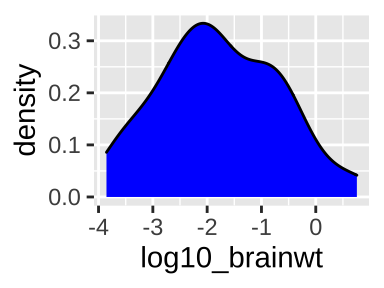Sometimes we prefer a smooth density plot to a histogram, as this can allow us to not get too distracted by a few bumps (on the other hand, we can also miss important variability, so be careful). We again map total_sleep onto the x aesthetic, but now use geom_density().

### 5.4.2 Two variables

With two variables, we want to highlight the association between them. In the plots below, we show that how this is presented can influence our biological interpretation and take-home messages.

#### Two categorical variables

With two categorical variables, we usually add color to a barplot to identify the second group. We can choose to

Below, we’ll make one of each of these graphs to look at this for the association between mammal order and diet, limiting our view to orders with five or more species with data. Which of these you choose depends on the message, story and details. For example, a filled barplot is nice because we can see proportions, but a bummer because we don’t get to see counts. The book advocates for mosaic plots, which I really like but skip here because they are a bit esoteric. Look into the ggmosaic package, and its vignette if you want to make one.

First, we process our data, making use of the tricks we learned in Handling data in R. To do so, we filter() for not NA diets, add_count() to see how many species we have in each order, and filter() for orders with five or more species with diet data.

# Data processing
msleep_data_ordervore <- msleep  %>%
filter(!is.na(vore))         %>%  # Only cases with data for diet
add_count(order)             %>%  # Find counts for each order
filter(n >= 5)                    # Lets only hold on to orders with 5 or more species with data
##### Two categorical variables: A stacked bar plot
ggplot(data = msleep_data_ordervore, aes(x = order,  fill= vore))+
geom_bar()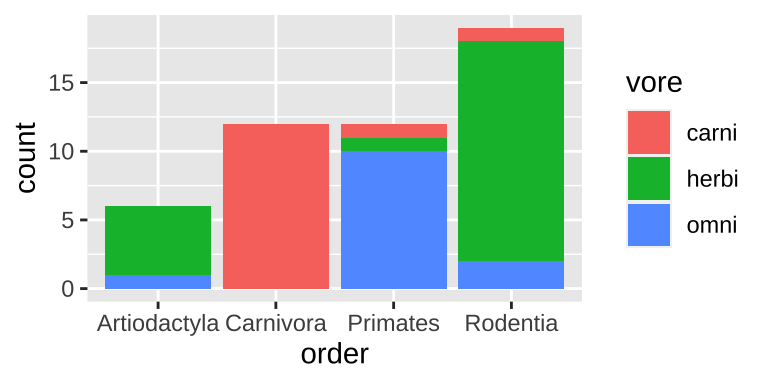Figure 5.8: A stacked bar chart.

Stacked barplots are best suited for cases when we’re primarily interested in total counts (e.g. how many species do we have data for in each order), and less interested in comparing the categories going into these counts. Rarely is this the best choice, so don’t expect to make too many stacked barplots.

##### Two categorical variables: A grouped bar plot
ggplot(data = msleep_data_ordervore, aes(x = order,  fill= vore))+
geom_bar(position = position_dodge(preserve = "single"))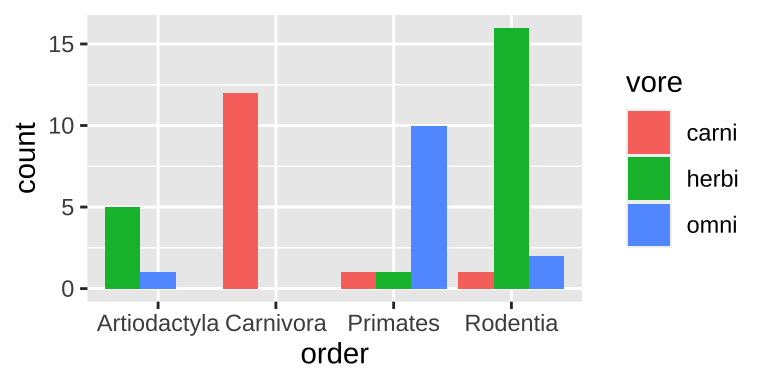Figure 5.9: A grouped bar chart.

Grouped barplots are best suited for cases when we’re primarily interested in comparing the categories going into these counts. This is often the best choice, as we get to see counts. However the total number in each group is harder to see in a grouped than a stacked barplot (e.g. it’s easy to see that we have the same number of primates and carnivores in Fig. 5.8, while this is harder to see in Fig. 5.9).

##### Two categorical variables: A filled bar plot
ggplot(data = msleep_data_ordervore, aes(x = order,  fill= vore))+
geom_bar(position = "fill")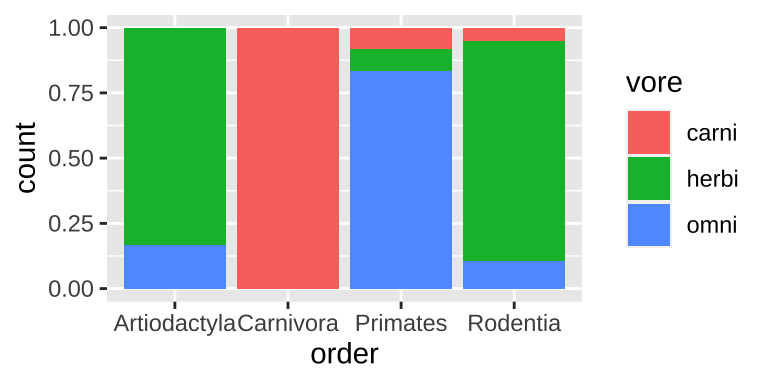Figure 5.10: A filled bar chart.

Filled barplots are much stacked barplots standardized to the same height. In other words, they are like stacked bar plots without their greatest strength. This is rarely a good idea, except for cases with only two or three options for each of numerous categories.

#### 5.4.2.1 One categorical and one continuous variable.

##### One categorical and one continuous variable: Multiple histograms

A straightforward way to show the continuous values for different categories is to make a separate histogram for each numerous distributions is to make separate histograms for each category using the geom_histogram() and facet_wrap() functions in ggplot.

msleep_data_ordervore_hist <- ggplot(msleep_data_ordervore, aes(x= log10_bodywt))+
geom_histogram(bins = 10)

msleep_data_ordervore_hist +
facet_wrap(~order, ncol = 1)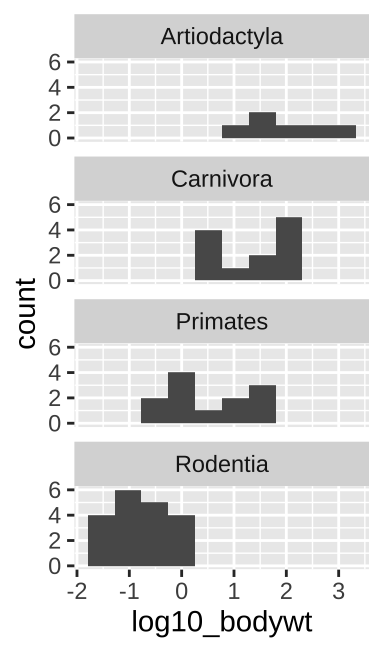Figure 5.11: Multiple histograms

When doing this, be sure to aid visual comparisons simple by ensuring there’s only one column. Note how Figure 5.11 makes it much easier to compare distributions than does Figure 5.12.

msleep_data_ordervore_hist +
facet_wrap(~order, nrow = 1)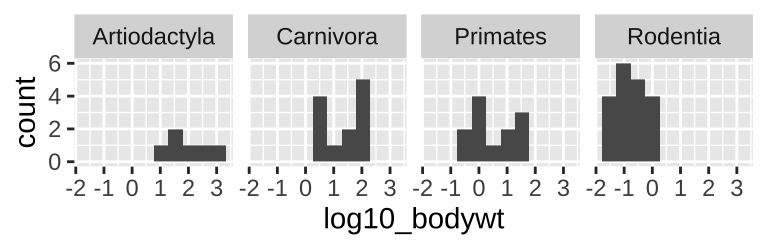Figure 5.12: Multiple histograms revisited

##### One categorical and one continuous variable: Density plots
ggplot(msleep_data_ordervore, aes(x= bodywt, fill = order))+
geom_density(alpha = .3)+
scale_x_continuous(trans = "log10")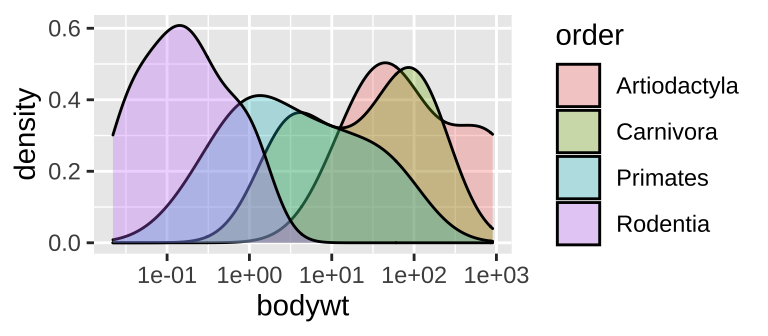Figure 5.13: A density plot

While many histograms can be nice, they can also take up a lot of space. Sometime we can more succinctly show distributions for each group with numerous density plots (geom_density()). While this can be succinct, it can also get too crammed, so have a look and see which display is best for your data and question.

##### One categorical and one continuous variable: Boxplots, jitterplots etc..

Histograms and density plots communicate the shapes of distributions, but we often hope to compare means and get a sense of variability.

• Boxplots (Figure 5.14A) summarize distributions by showing all quartiles – often showing outliers with points. e.g. ggplot(aes(x = order, y = bodywt)) + geom_boxplot().

• Jitterplots (Figure 5.14B) show all data points, spreading them out over the x-axis. e.g. ggplot(aes(x = order, y = bodywt)) + geom_jitter().

• We can combine both to get the best of both worlds (Figure 5.14C). e.g. ggplot(aes(x = order, y = bodywt)) + geom_boxplot() + geom_jitter().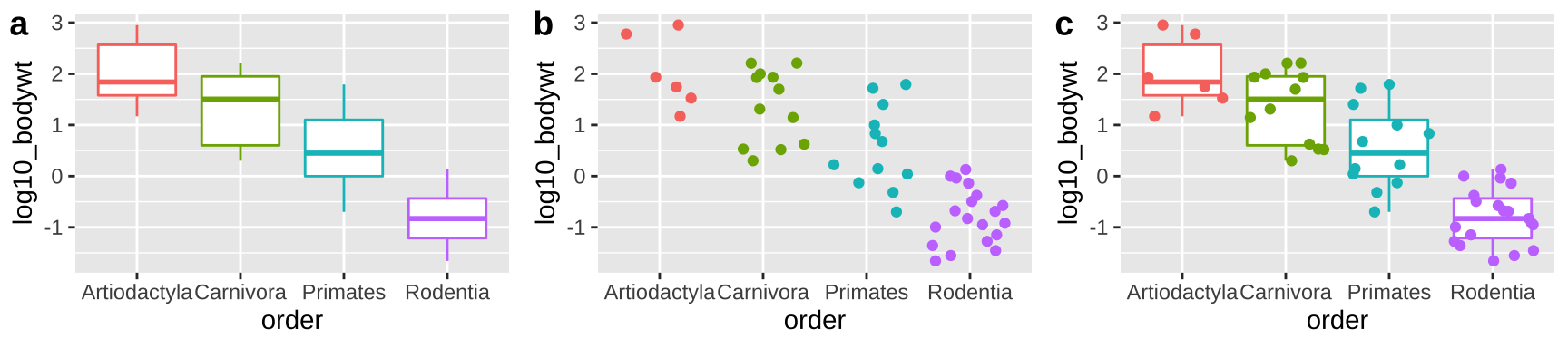Figure 5.14: Boxplots, jitterplots and a combination

#### 5.4.2.2 Two continuous variables

ggplot(msleep_data_ordervore, aes(x = log10_bodywt, y = log10_brainwt))+
geom_point()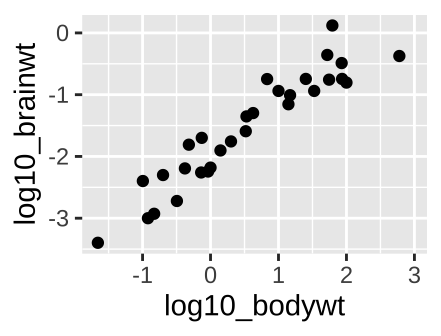Figure 5.15: A scatterplot.

With two continuous variables, we want a graph that visually display the association between them. A scatterplot displays the explanatory variable n the x-axis, and the response variable on the y-axis. The scatterplot in figure 5.15, shows a clear increase in brain size with body size across mammal species when both are on $$log_{10}$$ scales.

### 5.4.3 lineplots

Sometime we want points connected by lines (i.e. a lineplot). Lineplots are most usefull when we are looking at trends over time (e.g. when the x-axis is time). In such cases, geom_line() is here for us! Below I first modify the lynx dataset – which describes the number of lynx trapped in Canada every year from 1821 to 1934 – to make it a tibble (don’t worry about this code).

canadian_lynx <- tibble(year = start(lynx): end(lynx),  lynx_trapped = c(lynx))
glimpse(canadian_lynx)
## Rows: 114
## Columns: 2
## $year <int> 1821, 1822, 1823, 1824, 1825, 1826, 1827, 1828, 1829, 183… ##$ lynx_trapped <dbl> 269, 321, 585, 871, 1475, 2821, 3928, 5943, 4950, 2577, 5…

We can now plot these data as a lineplot

ggplot(canadian_lynx, aes(x  = year, y = lynx_trapped))+
geom_line()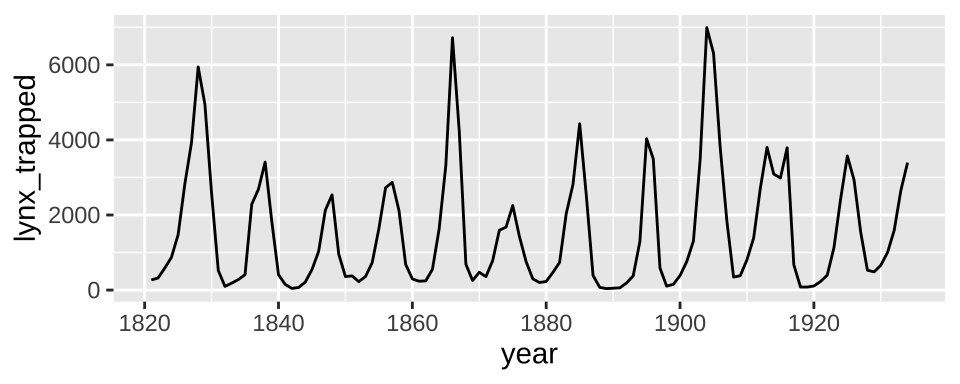Figure 5.16: A lineplot for the lynx dataset

ggplot(canadian_lynx, aes(x  = year, y = lynx_trapped))+
geom_point()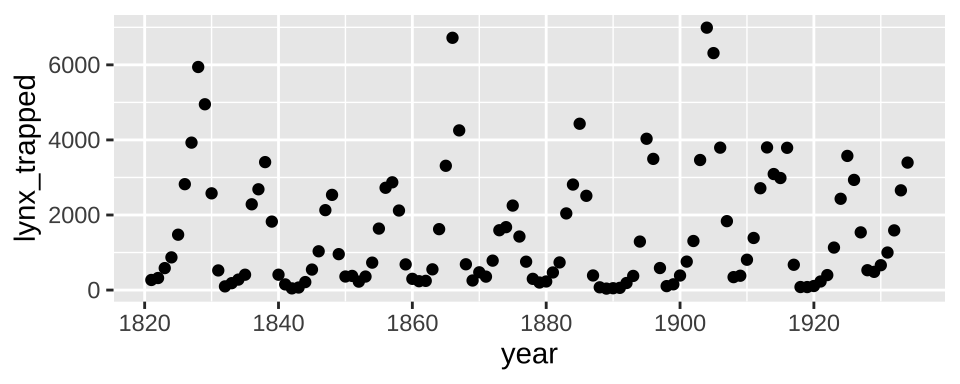Figure 5.17: A scatterplot that should be a lineplot for the lynx dataset

As you can see, the lineplot more clearly show the trends than does the scatterplot.

### 5.4.4 More dimensions

ggplot(msleep_data_ordervore,
aes(x = log10_bodywt, y = log10_brainwt, color = vore, shape = order))+
geom_point()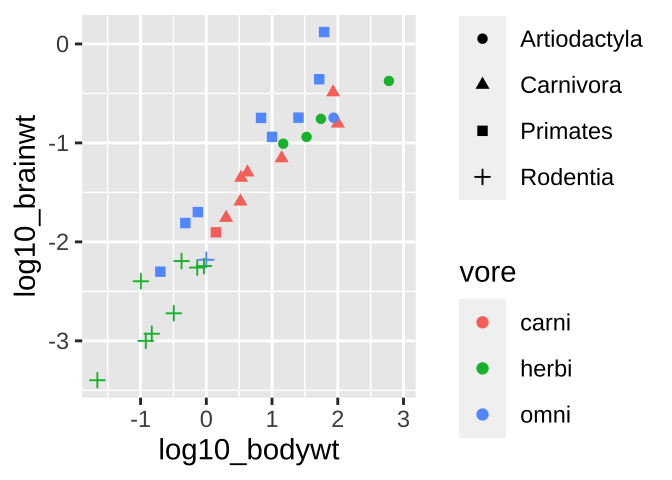Figure 5.18: Colored scatterplot

What if we wanted to see even more? Like let’s say we wanted to know if we found a similar relationship between brain weight and body weight across orders and/or if this relationship was mediated by diet. We can pack more info into these plots.

⚠️ Beware, sometimes shapes are hard to differentiate.⚠️ Facetting might make these patterns stand out.

ggplot(msleep_data_ordervore, aes(x = log10_bodywt, y = log10_brainwt, color = vore))+
geom_point()+
facet_wrap(~order, nrow = 1)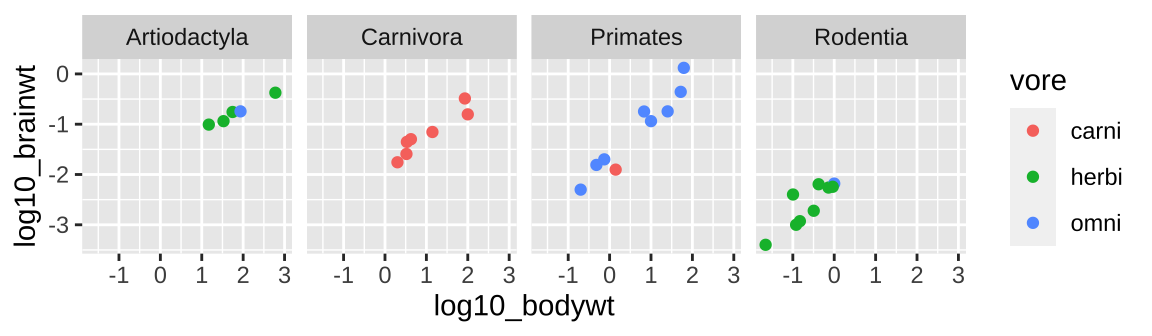### 5.4.5 Interactive plots with the plotly package

Often when I get a fresh data set I want to know a bit more about the data points (to e.g. identify outliers or make sense of things). The plotly package is super useful for this, as it makes interactive graphs that we can explore.

# install.packages("plotly") first install plotly, if it's not installed yet
library(plotly) # now tell R you want to use plotly
# Click on the plot below to explore the data!

big_plot <- ggplot(msleep_data_ordervore,
aes(x = log10_bodywt, y = log10_brainwt,
color = vore, shape = order, label = name))+
geom_point()

ggplotly(big_plot)

#### Decoration vs information

ggplot(msleep_data_ordervore, aes(x = log10_bodywt, y = log10_brainwt))+
geom_point(color = "firebrick", size = 3, alpha = .5)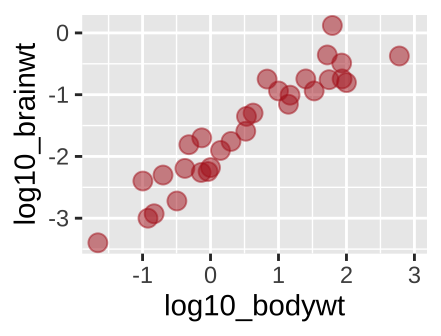Figure 5.19: A new scatterplot

We have used the aes() argument to provide information. For example, in Figure 5.15 we used color to show a diet by typing aes(…, color = vore). But what if we just want a fun color for data points. We can do this by specifying color outside of the aes argument. Same goes for other attributes, like size etc, or transparency (alpha)…

## 5.5 ggplot Assignment

Watch the video about getting started with ggplot

Complete RStudio’s primer on data visualization basics.

Make three plots from the mpg data and describe the patterns they highlight.

Fill out the quiz on canvas, which is very similar to the one below.

## 5.6 ggplot2 review / reference

### 5.6.1 ggplot2: cheat sheet

There is no need to memorize anything, check out this handy cheat sheet!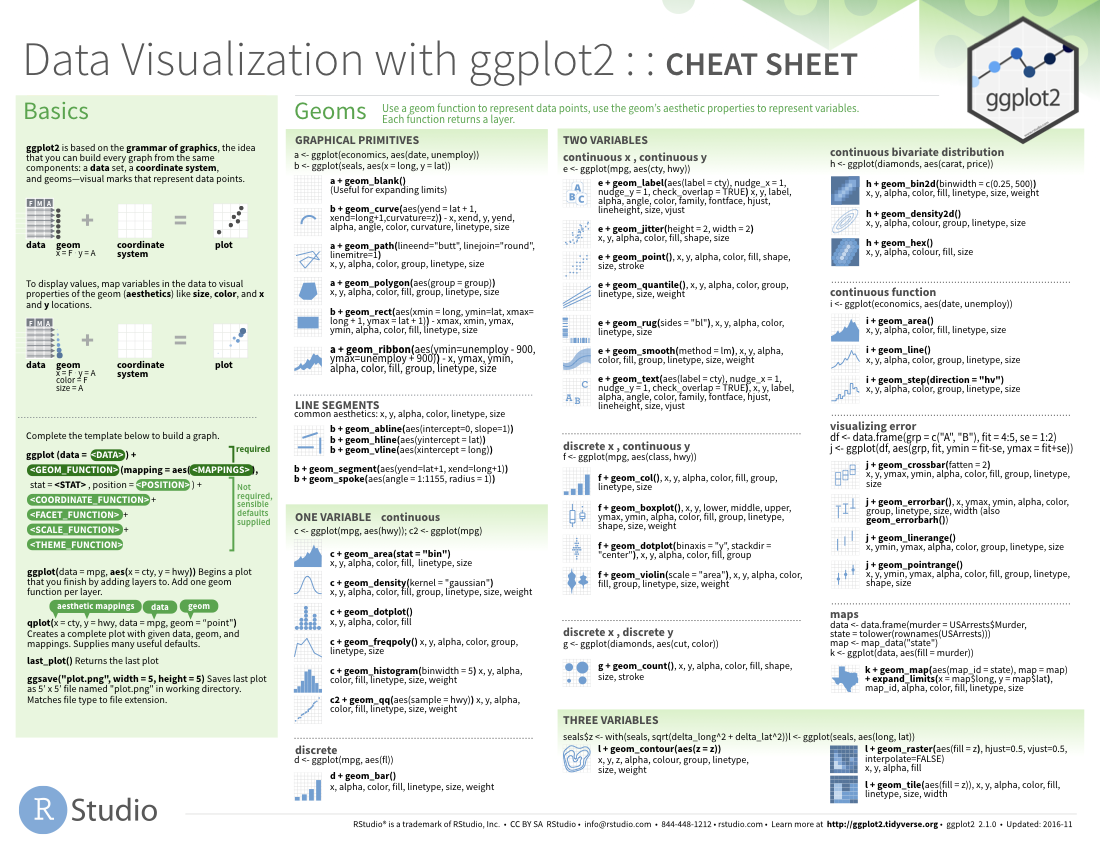#### 5.6.1.1 ggplot2: common functions, aesthetics, and geoms

##### The ggplot() function
• Takes arguments data = and mapping =.
• We usually leave these implied and type e.g. ggplot(my.data, aes(...)) rather than ggplot(data = my.data, mapping = aes(...)).
• We can pipe data into the ggplot() function, so my.data %>% ggplot(aes(…)) does the same thing as ggplot(my.data, aes(…)).
##### Arguments for aes() function

The aes() function takes many potential arguments each of which specifies the aesthetic we are mapping onto a variable:

###### x, y, and label:
• x: What is shown on the x-axis.

• y: What is shown on the y-axis.

• label: What is show as text in plot (when using geom_text())

##### Faceting

Faceting allows us to use the concept of small multiples to highlight patterns.

For one facetted variable: facet_wrap(~ <var>, nocl = )

For two facetted variable: facet_grid(<var1>~ <var2>), where one is shown by rows, and is shown by columns.

### References

Grolemund, Garrett, and Hadley Wickham. 2018. “R for Data Science.”
Healy, Kieran. 2018. Data Visualization: A Practical Introduction. Princeton University Press.
Tufte, Edward R. 1983. The Visual Display of Quantitative Information. pub-gp:adr: pub-gp.
Whitlock, Michael C, and Dolph Schluter. 2020. The Analysis of Biological Data. Third Edition.
———. 2016. Ggplot2: Elegant Graphics for Data Analysis. Springer-Verlag New York. https://ggplot2.tidyverse.org.